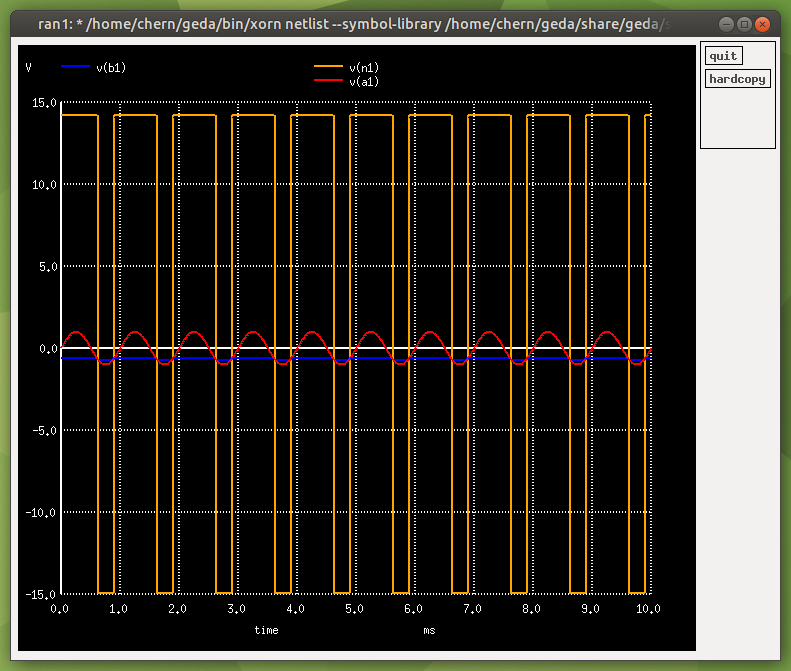# Comparator

A basic comparator is shown below (in practice, a comparator will have some hysteresis, usually implemented with a Schmitt Trigger). We use ground (0V) for the reference at the inverting input. Therefore when the signal at the non-inverting input is greater than 0V, the output is high. When the signal at the non-inverting input is less than 0V, the output is low.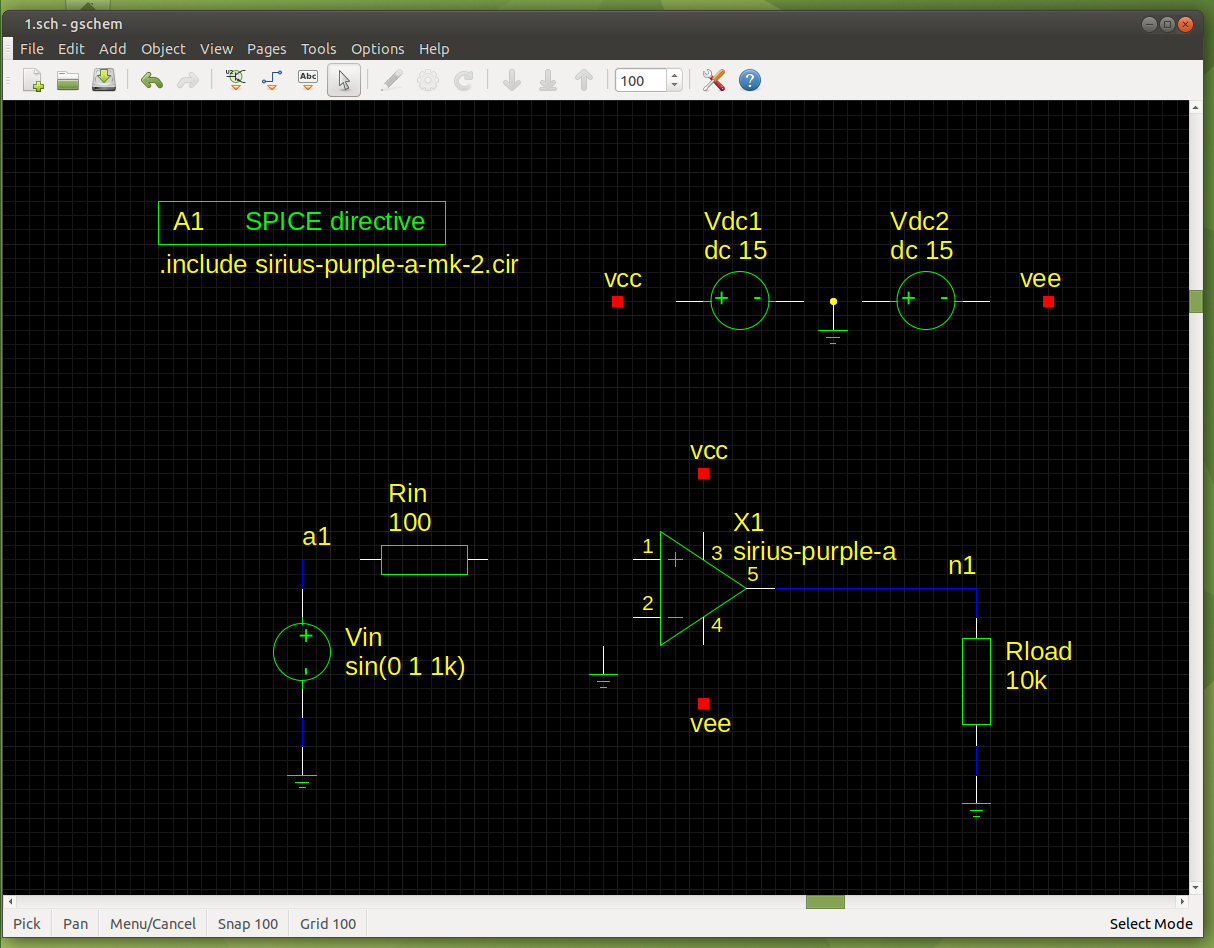Transient Analysis:

`tran 0.01m 10m`
`plot v(a1) v(n1)`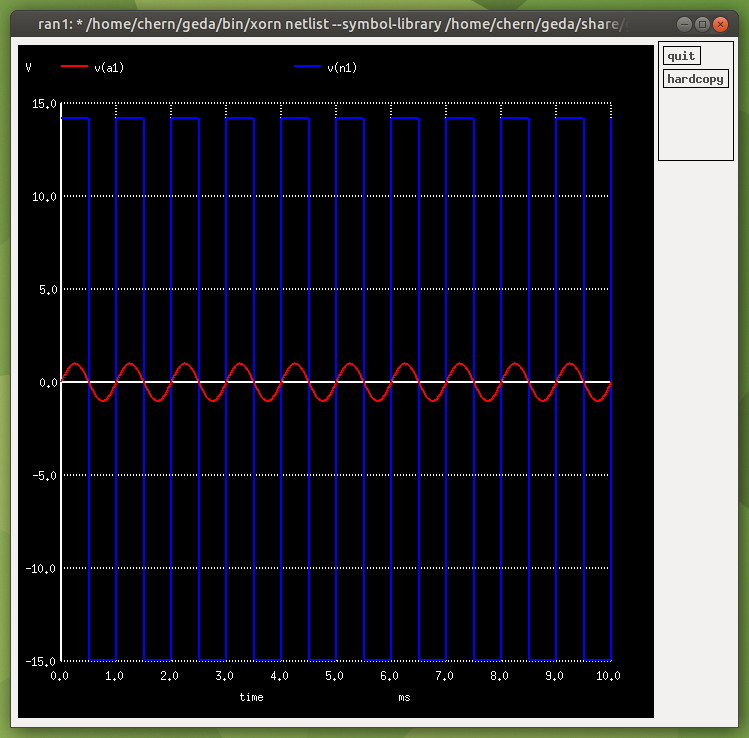To make the output inverted, just swap the inputs around: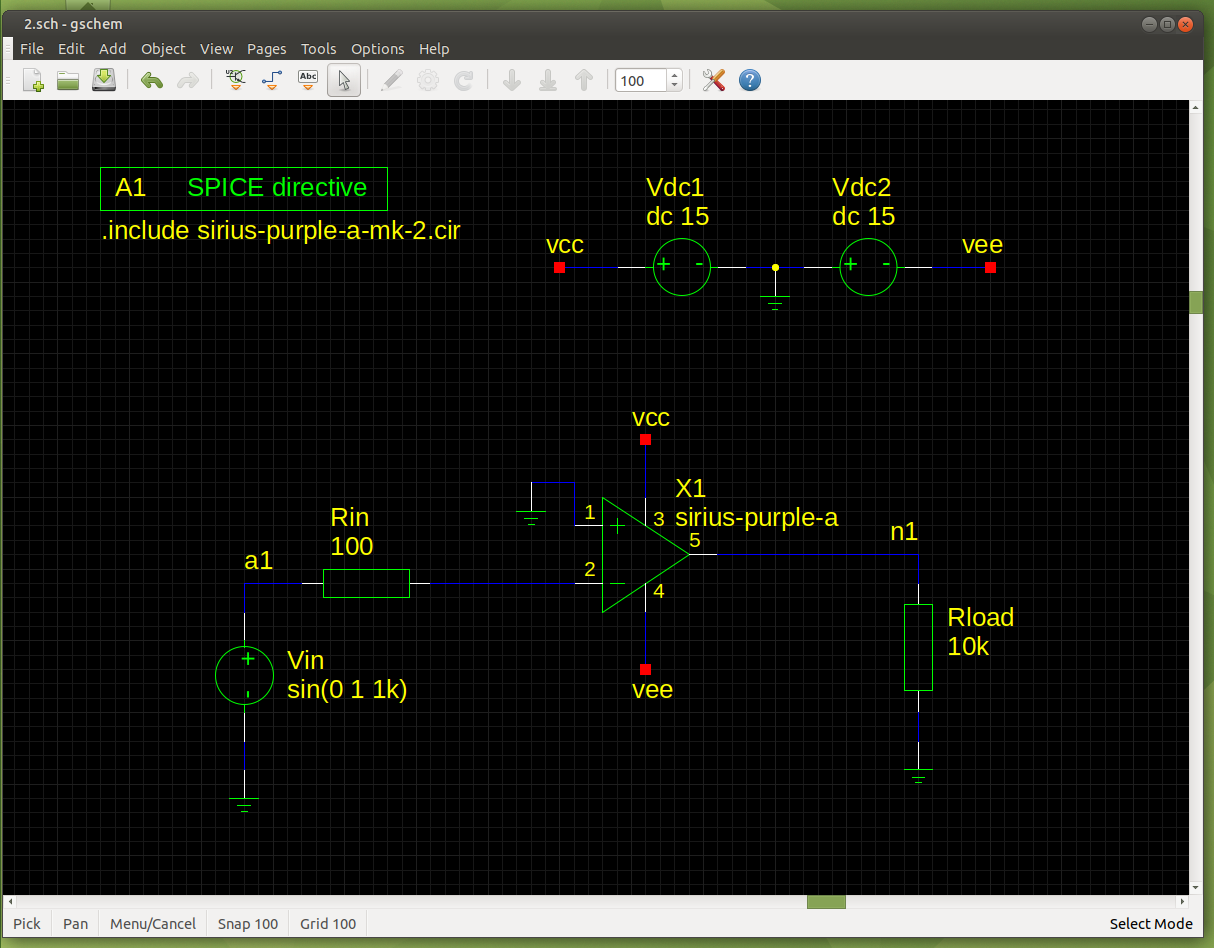Transient Analysis: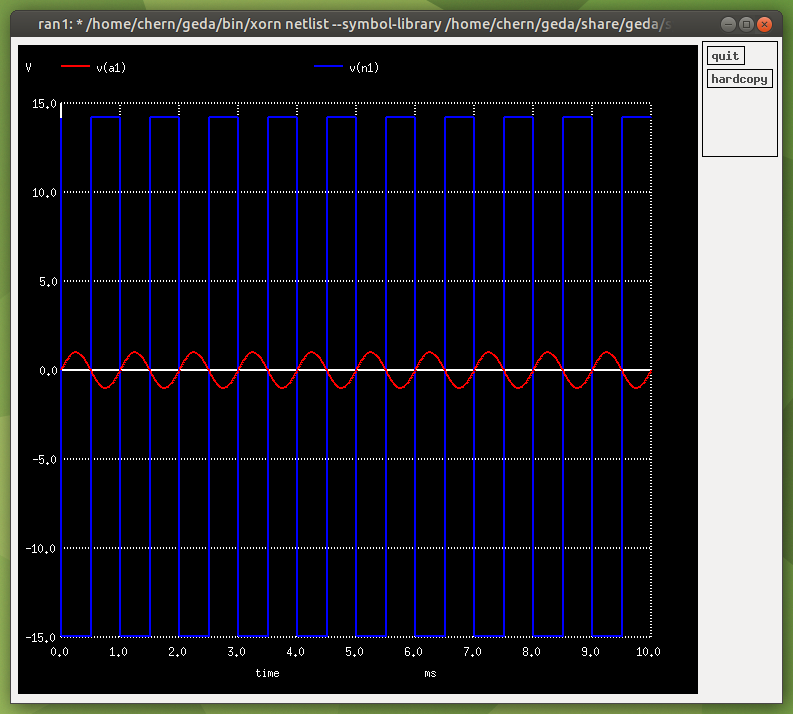You can use a voltage divider to set the reference voltage: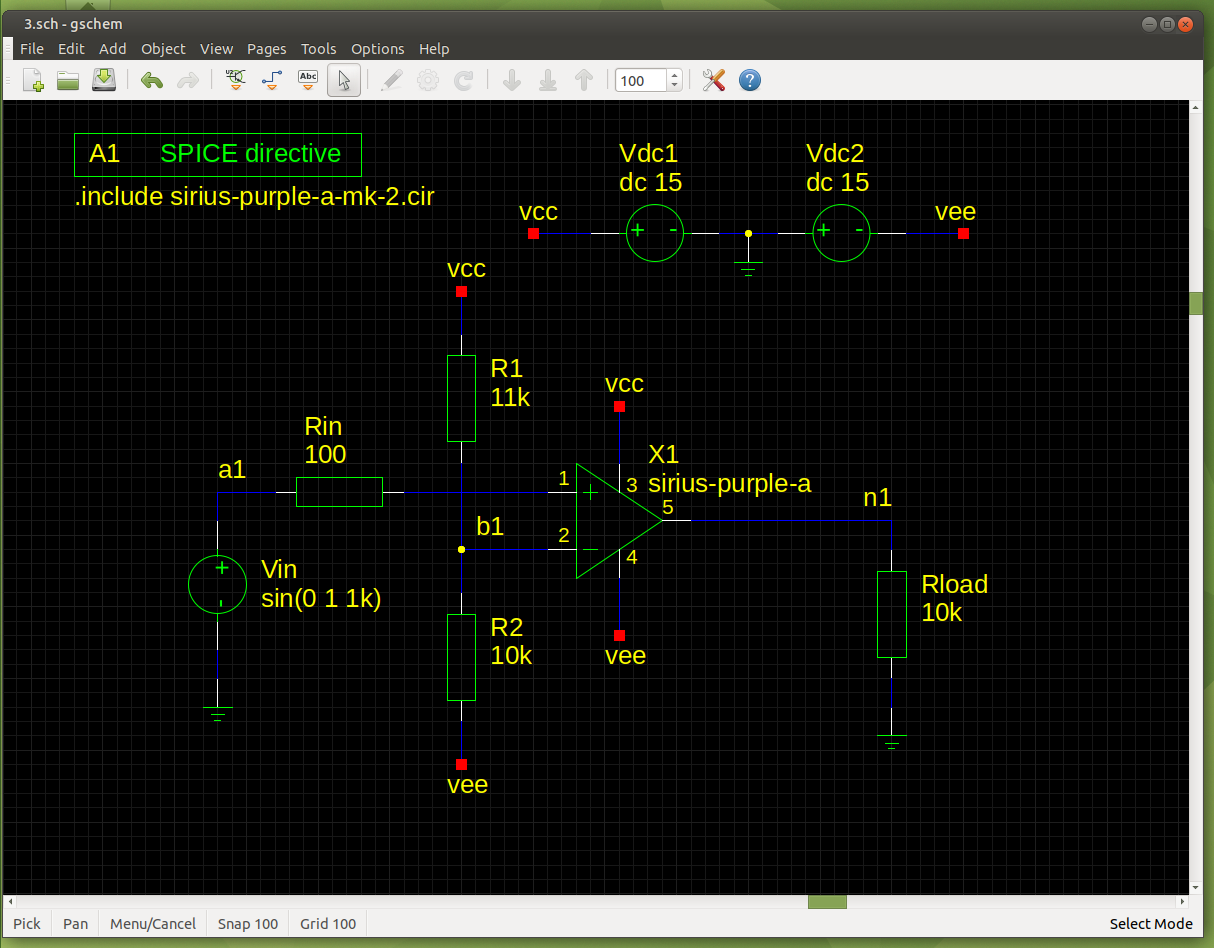Transient Analysis: